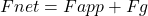## What is the centripetal force for a roller coaster if the mass is 10 kg and the normal force is 25 N?

Question

What is the centripetal force for a roller coaster if the mass is 10 kg and the normal force is 25 N?

in progress 0
7 months 2021-08-01T16:19:59+00:00 1 Answers 10 views 0

## Answers ( )

Fc = 123 Newton

Explanation:

Net force can be defined as the vector sum of all the forces acting on a body or an object i.e the sum of all forces acting simultaneously on a body or an object.

Mathematically, net force is given by the formula;Where;

Fnet is the net force.

Fapp is the applied force.

Fg is the force due to gravitation.

Given the following data;

Normal force = 25N

Mass = 10kg

To find the centripetal force;

From the net force, we have the following formula;

Fc = N + mg

Where;

Fc is the centripetal force.

N is the normal force.

mg is the the weight of the object.

Substituting into the formula, we have;

Fc = 25 + 10(9.8)

Fc = 25 + 98

Fc = 123 Newton